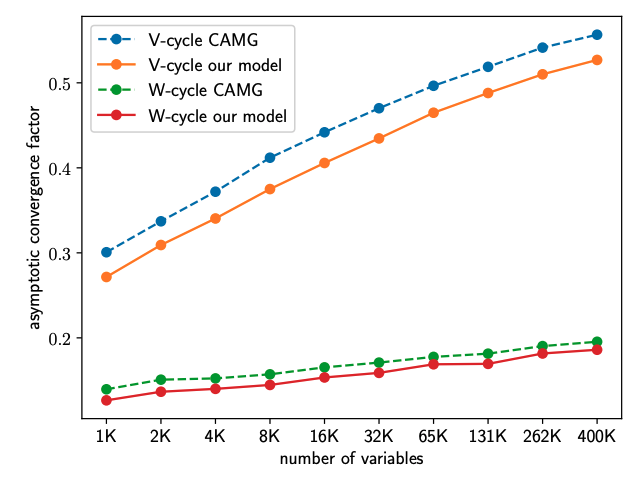# Learning Algebraic Multigrid Using Graph Neural NetworksEfficient numerical solvers for sparse linear systems are crucial in science and engineering. One of the fastest methods for solving large-scale sparse linear systems is algebraic multigrid (AMG). The main challenge in the construction of AMG algorithms is the selection of the prolongation operator -- a problem-dependent sparse matrix which governs the multiscale hierarchy of the solver and is critical to its efficiency. Over many years, numerous methods have been developed for this task, and yet there is no known single right answer except in very special cases. Here we propose a framework for learning AMG prolongation operators for linear systems with sparse symmetric positive (semi-) definite matrices. We train a single graph neural network to learn a mapping from an entire class of such matrices to prolongation operators, using an efficient unsupervised loss function. Experiments on a broad class of problems demonstrate improved convergence rates compared to classical AMG, demonstrating the potential utility of neural networks for developing sparse system solvers.

## Authors

Ilay Luz (Weizmann Institute of Science)
Meirav Galun (Weizmann Institute of Science)
Ronen Basri (Weizmann Institute of Science)